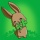Home Communities
IT Knowledge
Inspiration
Languages
EN

# JavaScript - random values from array with probability (different probability per item)

9 points
Created by:JustMike
30890

In this short article, we would like to show how in JavaScript, get random items from an array using different randomization probabilities per item.

The main idea used in the below example to randomize items with different probabilities is to create an array that represents items distribution - items are repeated many times to affect randomization.

Quick solution:

``````// ONLINE-RUNNER:browser;

const createDistribution = (array, weights, size) => {
const distribution = [];
const sum = weights.reduce((a, b) => a + b);
const quant = size / sum;
for (let i = 0; i < array.length; ++i) {
const limit = quant * weights[i];
for (let j = 0; j < limit; ++j) {
distribution.push(i);
}
}
return distribution;
};

const randomIndex = (distribution) => {
const index = Math.floor(distribution.length * Math.random());  // random index
return distribution[index];
};

// Usage example:

const array =   ['John', 'Chris', 'Ann'];  // used values in radomization
const weights = [  0.5 ,    0.2 ,  0.3 ];  // specific items probability (5 + 2 + 3 = 10)

const distribution = createDistribution(array, weights, 10);  // 10 - describes distribution array size (it affects on precision)

for (let i = 0; i < 10; ++i) {
const index = randomIndex(distribution);
console.log(array[index]);  // random value located in the array
}``````

Example output:

``````John
Chris
Ann
Chris
Chris
Ann
John
John
Ann
John``````

## Alternative titles

Join to our subscribers to be up to date with content, news and offers.